## Saturday, February 14, 2009

### Irodov Problem 1.275To understand what is happening let us compare the two cases i) when the rod is free and not pivoted at point P versus ii) when it is pivoted at point P as in this problem.

In case of the free rod, the rod would spin about the CG and the CG of the rod would move linearly forward. The angular velocity w of the rod after the collision would depend on how far near the end of the rod the point mass collides. The closer it is to the center, the less the angular velocity would be. If the point mass were to hit exactly at the center, the angular velocity would be w=0. Immediately, after the collision the net velocity of point P would be the sum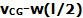.
If the point mass hits close to the center then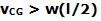and so the net velocity of P will be to the right (in the direction of CG). However, if the point mass hits closer to end of the rod, thenand the net velocity of P will in the opposite direction of that of CG (to the left).

With the above discussion in mind, let us consider the pivoted case now. As soon as the rod is impinged upon by the point mass close to one of its ends, point P of the rod has a tendency to move backwards (to the left) as discussed in the free rod case. However, the pivot at point P does not allow this. To prevent, this motion of point P to the left, the pivot exerts a reaction force
F to the right and arrests point P at the same location. This, force F, is external to the (rod + point mass) system and so it results in an increase in momentum on the (rod+point mass system). This explains why, the net momentum of the (rod + point mass) system will increase if the point mass strikes at the end.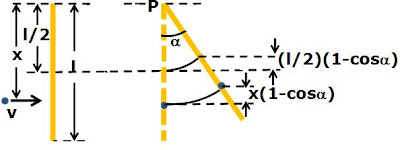We shall solve the problem generally with the point mass striking at a distance x from the pivoted end since this will help solve all the parts of the problem at the same time.

a) Since this force F acts at point P, there is no torque generated during the collision process about an axis passing through P (since moment arm is 0). Consequently, the angular momentum of the (point mass + rod) system is conserved just before and after the collision. Let the initial velocity of the point mass be v. The initial angular momentum of the point mass m about the axis through P perpendicular to the plane of motion of rod and point mass is given by mvx. After the point mass get embedded into the rod, the moment of inertia of the (rod + point mass) about the axis through P is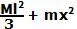(see the list of moment of inertias). Let the angular velocity of the (point mass + rod) immediately after collision be w. Since the angular momentum just before and after collision must be conserved, we have,After this point, the point mass and the rod together as one body, will swing upwards, rotating about the axis through P. As a consequence, they will gain potential energy at the cost of loosing kinetic energy. At the higher point of the accent, the entire kinetic energy will become potential energy. The initial kinetic energy of the (point mass + rod) system is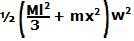. At the highest position of the swing the rod has gained a potential energy ofsince its CG raised by a height ofas shown in the figure. Similarly, the point mass gains a potential energy of. Hence, from conservation of energy we have,From (1) and (2) we can calculate the initial velocity v as,If M>>m and x= l then (3) becomes,(b) As discussed at the beginning of the problem the momentum of the (point mass + rod) system will change before and after impact, due to the reaction force from the pivot. The initial momentum is clearly mv. The mometum of the rod after the impact is the velocity of the CG of the rod times the mass of the rod given by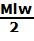. The momentum of the point mass is mwx. Thus, the total momentum just after impact is given by,(c) From (5) it is clear that when 3x=2l or x = 2l/3, the change in momentum will be 0.